ChemTalk

# Titration Curves & Equivalence Point Calculations## Core Concepts

In this tutorial, you will learn about titration curves, titration analysis and the steps required to perform one. You will also learn about equivalence points and endpoints, and titration calculations.

## Introduction to Titration

### What is the definition of titration?

Titration is a laboratory technique used to determine the concentration of a solution by reacting it with a known volume and concentration of another solution. This is done by carefully measuring out a specific volume of the first solution, called the titrant, and adding it to the second solution, called the analyte, until the reaction is complete. The point at which the reaction is complete is called the endpoint, and it can be detected using a variety of methods, such as a color change or a change in electrical conductivity. The concentration of the analyte solution can then be calculated based on the volume and concentration of the titrant that was used.

Titration is a useful tool for determining the concentration of a wide range of substances, including acids, bases, and other chemicals. Scientists employ titration to assess the quantity of a constituent, or analyte, of a given sample. They accomplish this by adding a known quantity of another substance, called a standard solution, to the sample.

To learn how to create a known concentration of a solution, check out this article on molarity. This standard solution contains the titrating reagent, or titrant. When titrant is added, it reacts with the analyte in a known proportion (according to the reaction equation) with the titrant, revealing its concentration and amount.A manual titration apparatus. The upper piece of glassware is called a burette, and is filled with titrant. The red and white plastic stopcock is used to meter the flow of titrant into the analyte solution below. Here, the analyte is slightly pink, indicating that the titration has just reached the endpoint, probably with phenolphthalein indicator. Photo by Kengksn, distributed under a CC-BY 2.0 license.

## Types of Titrations

### 1. Acid-Base Titrations

This kind of titration determines the concentration of an acid or the concentration of a base. The former investigation uses a basic standard solution to neutralize the sample, while the latter uses an acidic standard solution. You can also determine the strength of the acid or base by viewing the shape of the titration curve.

### 2. Redox (Oxidation-Reduction) Titrations

This type of titration involves a redox chemical reaction between the analyte and the titrant, during which these substances transfer electrons.

### 3. Precipitation Titrations

This titration variety uses the formation of a precipitate to signal the completion of the reaction between the analyte and the titrant.

### 4. Complexometric Titrations

During this type of titration, a larger, undissociated complex forms. This generates less room for interpretation errors than normal precipitation titrations.

### 5. Thermometric Titrations

This type measures the temperature of the solution to determine when the reaction is complete. It relies on the heat generated or absorbed during the reaction of the analyte and titrant.

## Equivalence Point versus Endpoint

But how do scientists determine the point at which chemically equivalent quantities of analyte and titrant exist in the solution, so that they can quantify the analyte? They look for an “equivalence point,” the point at which enough titrant has combined with the analyte to neutralize it. At this specific point, the amount of titrant in the system reveals the amount of analyte in the system; the moles of both species equal one another.

However, this equivalence point differs from the “endpoint” of a titration analysis. The endpoint indicates the end of the reaction; it denotes the amount of reactant titrant needed to facilitate a complete chemical reaction with the reactant analyte. A color change in the system of interest signals that it has reached this endpoint. Materials called “indicators,” which undergo these color changes, can be added to the system to designate the endpoint.

Different indicators exhibit different endpoints due to their varying chemical compositions. Scientists tend to choose indicators whose endpoints roughly equal their equivalence points. When this occurs, the color transition denotes both the endpoint and the equivalence point, revealing the amount of titrant needed to equal the amount of analyte and thus the quantity of analyte in the system. Some substances, such as polyprotic acids, possess multiple equivalence points, but for a given indicator there is generally only one endpoint.

Below is a chart summarizing the characteristics differentiating equivalence points and endpoints:

## Titration Curves

Titration curves provide graphical depictions of titration analyses. Scientists first measure a property of the analyte solution (usually pH for acid-base titrations). Then, they plot this property against the volume of the titrant added.

Using a titration curve, scientists can often identify an endpoint closer to the equivalence point, compared with using an indicator to find the endpoint.

In addition to pH, several other properties can reveal the endpoint of a reaction. These include the absorptivity, temperature, conductivity, or electrical current generated. Some of these are only applicable to certain types of reactions.

### Acid-Base Titration Curves

Acid-base titration, the most frequently employed method of titration, adheres to certain curve trends established by the acid and the base involved. The pH of the solution at the equivalence point depends on the characteristics of the analyte and the titrant. The following combinations produce:

• Strong Acid combined with Strong Base: equivalence point at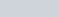• Weak Acid combined with Strong Base: equivalence point at• Strong Acid combined with Weak Base: equivalence point atBelow is an Acid-Base Titration curve used to quantify the oxalic acid analyte using NaOH as a titrant. The curve consists of a plot displaying pH. The equivalence point of a system occurs during a vertical increase in its pH values, while the endpoint of a visual indicator tends to occur during the last slight increase in pH value. The endpoint shown below is based on the indicator, and cannot be determined from the curve itself.

## How to Perform a Simple Titration

The following steps describe the methods involved in a titration analysis using a visual indicator:

1. Fill your burette with titrant solution, using the graduations on the instrument to precisely gauge the amount added.
2. Clamp the burette to your burette stand. Make sure the stand clamps adhere tightly to the burette to minimize movement during the analysis.
3. Fill a beaker with your analyte solution, again ensuring precise measurements using the graduations on the glassware
4. Using a pipette or dropper, add a few drops of indicator to the analyte solution. The type and amount of indicator required will depend on your titrant and analyte.
5. Next, agitate your analyte solution by placing it on a magnetic stir plate. This thoroughly mixes the components.
6. Place the beaker, containing the analyte, directly under the burette, containing the titrant. Gently turn the stopcock to allow the titrant to drip from the burette to the beaker.
7. Continue adding titrant solution to analyte solution until you observe a color change, indicating the endpoint of the solution. If the indicator’s endpoint equals its equivalence point, you can infer that the amount of analyte in the system equals the amount of titrant at this point.
8. Determine how much titrant you added to the analyte by subtracting the final volume in the burette from the starting volume.
9. Quantify the amount of analyte in the system using the amount of titrant added; these amounts should be equivalent.

## Titration Calculations

Titration calculations generally involve this equation: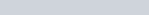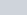is the molarity of the acid, while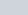is the molarity of the base.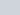and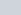are the volumes of the acid and base, respectively. You want to measure the volumes at the endpoint of the titration, when the acid and base have neutralized each other. Generally the two volumes are known, and the molarity of the acid or the base is known, leaving only one variable left to solve for. For example, if the concentration of the base in unknown, then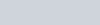.

## Automatic Titration Apparatus

Although the example discussed above is a manual titration, there are also many automatic titrators available today. These can often inject far more precise amounts of titrant than a standard burette would, and they can reduce waste by using extremely small quantities of reagents. Below is an image of a standard automatic titrator, which shows the physical apparatus as well as the software interface displaying a titration curve in the lower left corner.

## Applications of Titration in Today’s World

This analytical technique has many applications, ranging from medical, to cosmetic, and even to environmental science.

In the food industry, workers use titration and equivalence points to determine the quantity of different salts, sugars, vitamins, and fatty acids in foods. Similarly, other major industries like cosmetics and cleaning use titration to add appropriate and safe concentrations of chemicals to their products. Pharmaceutical companies rely on titration during the medication developing process. Even medical labs use this type of analysis to study blood and urine samples from patients.

In addition to industry, there are many uses for titration in academic research. For one, environmental chemists conduct titrations using rainwater, melted snow, and other liquids to assess their composition. For analysis of water content in an analyte, there is a special technique known as Karl-Fischer titration. In these and many other fields, titration also serves to determine the exact concentration of reagents, a process known as standardization.

As you can see, titration has an astounding range of uses and applications. As a result, it is one of the most frequently conducted methods of chemical analysis worldwide, both in the classroom and in the workplace.

## Titration Calculation Practice Problems

Problem 1

You have a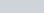solution of acetic acid. You add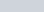of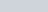NaOH to reach the equivalence point of the titration. What is the concentration of the acetic acid solution?

Acetic acid and NaOH react according to the following equation: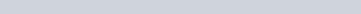Problem 2

You plan to titrate a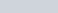solution of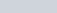H2O2 usingKMnO4. What volume of KMnO4 solution do you predict you will add?

H2O2 and KMnO4 react according to the following equation: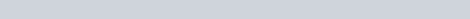## Titration Calculation Practice Problem Solutions

1: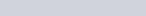2: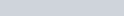Amazing facts about titration analysis, including the types, components, curves, and steps of titrations